Download Presentation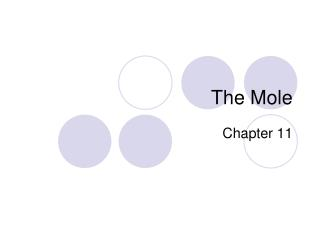The Mole

# The Mole - PowerPoint PPT Presentation

The Mole. Chapter 11. Particles Names. Atoms, formula units (ionic compounds), molecules (covalent compounds). Counting units. 1mole = 6.02 x 10 23 particles. Mole to Particle Conversions. How many molecules of water are there in 2.4 moles of water?I am the owner, or an agent authorized to act on behalf of the owner, of the copyrighted work described.
Download Presentation## The Mole

An Image/Link below is provided (as is) to download presentation

Download Policy: Content on the Website is provided to you AS IS for your information and personal use and may not be sold / licensed / shared on other websites without getting consent from its author.While downloading, if for some reason you are not able to download a presentation, the publisher may have deleted the file from their server.

- - - - - - - - - - - - - - - - - - - - - - - - - - E N D - - - - - - - - - - - - - - - - - - - - - - - - - -
Presentation Transcript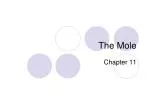### The Mole

Chapter 11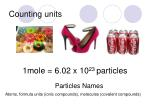Particles Names

Atoms, formula units (ionic compounds), molecules (covalent compounds)

Counting units

1mole = 6.02 x 1023 particles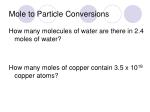Mole to Particle Conversions

How many molecules of water are there in 2.4 moles of water?

How many moles of copper contain 3.5 x 1018 copper atoms?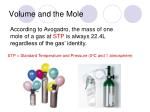Volume and the Mole

According to Avogadro, the mass of one mole of a gas at STP is always 22.4L regardless of the gas’ identity.

STP = Standard Temperature and Pressure (0oC and 1 atmosphere)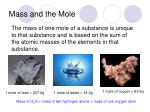Mass and the Mole

The mass of one mole of a substance is unique to that substance and is based on the sum of the atomic masses of the elements in that substance.

1 mole of copper = 63.6g

1 mole of lead = 207.2g

1 mole of water = 18.0g

Mass of H2O = mass of two hydrogen atoms + mass of one oxygen atom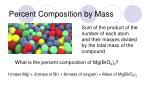Percent Composition by Mass

Sum of the product of the number of each atom and their masses divided by the total mass of the compound

What is the percent composition of Mg(BrO4)2?

1(mass Mg) + 2(mass of Br) + 8(mass of oxygen) = Mass of Mg(BrO4)2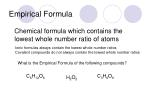Empirical Formula

Chemical formula which contains the lowest whole number ratio of atoms

Ionic formulas always contain the lowest whole number ratios

Covalent compounds do not always contain the lowest whole number ratios

What is the Empirical Formula of the following compounds?

C6H12O6

C3H9O6

H2O2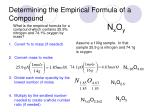Determining the Empirical Formula of a Compound

NxOy

What is the empirical formula for a compound which contains 25.9% nitrogen and 74.1% oxygen by mass?

Assume a 100g sample. In that sample 25.9 g is nitrogen and 74.1g is oxygen

• Covert % to mass (if needed)
• Convert mass to moles
• Divide each molar quantity by the lowest number of moles
• Multiply by the smallest number needed to create a whole number ratio (if needed)

N1.85O4.63

N1O2.5

N2O5

N1x2O2.5x2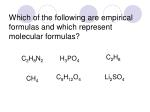Which of the following are empirical formulas and which represent molecular formulas?

C2H8

C2H8N2

H3PO4

C8H12O4

Li2SO4

CH4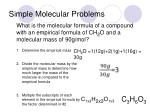Simple Molecular Problems

What is the molecular formula of a compound with an empirical formula of CH2O and a molecular mass of 90g/mol?

• Determine the empirical mass
• Divide the molecular mass by the empirical mass to determine how much larger the mass of the molecule is compared to the empirical formula
• Multiple the subscripts of each element in the empirical formula by the factor determined in #2

CH2O =1(12g)+2(1g)+1(16g) = 30g

C3H6O3

C1x3H2x3O1x3Longer Molecular Problems
• Determine the molecular formula
• Given the molecular mass, determine the molecular formula

Determine the molecular formula of a compound that has a mass of 34g and contains 94.1% oxygen and 5.9% hydrogen by mass.